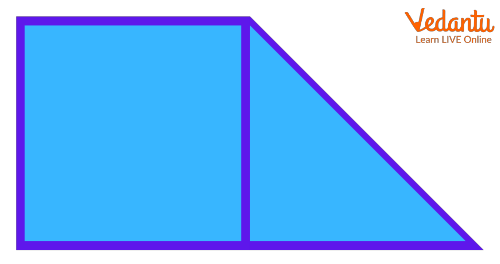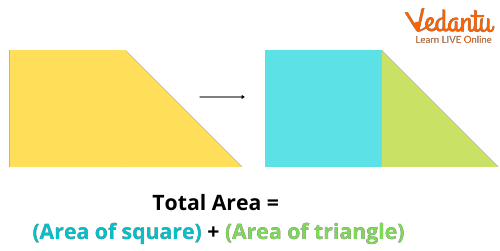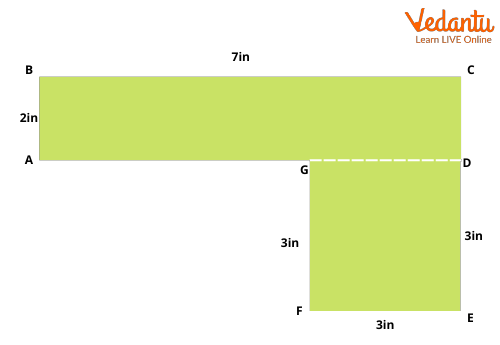Courses
Courses for Kids
Free study material
Free LIVE classes
More

# Composite FigureLIVE
Join Vedantu’s FREE Mastercalss

## Simple Ways to Teach Composite Figure to Kids

As kids start progressing in their classes, they are introduced to various new concepts in every subject. They are introduced to vast literature, history, political sciences and science. Science, especially mathematical operations, are very interesting and can be used to explain the cause and functioning of almost all natural phenomena. One such concept introduced in mathematics is the introduction of composite figures. By understanding what is a composite figure, kids will be able to know the measurement and geometry of the things around them. Needless to say, learning new things and doing experiments is fun. This article is aimed to help kids, parents and teachers to create a precise understanding of the composite figures. The article mentions the definition and examples of composite figures for a better understanding of the concept.

### What is a Composite Figure?

The composite figure can be defined as a shape that constitutes more than two-dimensional shapes. When deconstructed, a composite shape is made up of a number of other shapes. A more technical definition of the term is based on the constituent shapes that make up the composite figure. The composite figure is a two-dimensional figure constructed up of basic two-dimensional shapes such as triangles, rectangles, circles, semi-circles, and so on. The examples of the composite figures are mentioned below for a better understanding.The above image is made up of a triangle and squareImage illustrating a parallelogram made up of two triangles

### How to Calculate the Area of the Composite Figures or Shapes?

As we have learnt about the definition of composite figures, let us look into the areas of the composite figure. As we know that simple geometric forms make up a composite figure, the area can be calculated by dividing the composite figure into basic, non overlapping figures to get its area. In simpler terms, the overall area can be calculated by adding the areas of the individual area of the geometric shapes. This method of calculation of area in mathematics is known as the additive method of area calculation. Now as we have learnt about the additive method let us look into the units that are used to represent the area. m2, cm2, in2 or ft2 are some of the common units used to represent the area. Mentioned below is an image that can be used to explain the additive method.Image illustrating the area of the composite figure

### Formulas to Calculate the Area of Different Shapes

Along with learning the additive method, we must also know the formulas for the calculation of the area of the various shapes that can make up a composite figure. The table mentioned below provides a formula to calculate the area of triangle, square, rectangle and so on.

 Name of the Shape Formulas Triangle Area of triangle = (1/2) × base × height. Square Area of square = (length)2 Rectangle Area of rectangle = length × breadth Parallelogram Area of parallelogram = base × height Trapezium Area of trapezium = (1/2) × (sum of lengths of parallel sides) × height Rhombus Area of rhombus = (1/2) × (product of diagonals)

### Solved Examples

Example 1- Calculate the area of a composite figure that is made up of a square and a triangle. The triangle has a base of 6 cm while the height of the triangle is 7 cm. The side of the square is 5 cm.

Solution- Since we know that to calculate the area of a composite figure we must add the area of the individual shape. The figure is made up of triangles and squares, so let us calculate the area of a triangle and square.

Area of the square- (length)2

Area of the square- 52 = 25

Area of triangle = (1/2) × base × height.

Area of triangle = [(1/2) × 6 × 7] = 21

Area of composite shape= area of triangle + area of square

Area of composite shape= 21 + 25

Area of composite shape= 46 cm2

Example 2- Find the area of the figure given belowSolution- Area of composite shape = Area of rectangle + area of the square

area of the rectangle ABCD = length × breadth

area of the rectangle ABCD = 7 × 2 = 14

Area of square = (length)2

Area of square = 32 = 9

Area of composite shape = 14+9 = 23 in2

In conclusion of the article, we have learnt about the definition of the composite figure and calculation of the area of the triangle. We hope that this discussion will help kids to better understand the concept of composite figures.

Last updated date: 28th Sep 2023
Total views: 139.2k
Views today: 2.39k

## FAQs on Composite Figure

1. Which formula is used to calculate the area of a triangle by Heron's method?

The area of the triangle can be calculated by  (1/2) × base × height. Another formula that is used to calculate the area is Heron's formula. According to it, area = $\sqrt{s (s-a) (s-b) (s-c)}$ where (s) represents = Perimeter/2 = (a + b + c)/2.  The a, b and c is the length of their sides.

2 How does increasing the dimension that makes up the composite affect the composite shape?

As the area of the composite figure depends on the area of constituent shape, if the dimensions of them are increased the area of the composite figure will also increase.